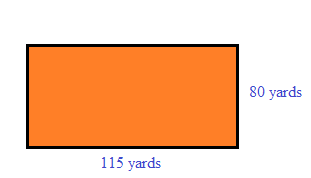### Home > ACC6 > Chapter 3 Unit 3 > Lesson CC1: 3.1.1 > Problem3-21

3-21.

Stacy exercises three days each week by walking around the soccer field near her home. The field is $80$ yards wide and $115$ yards long.

1. Draw a diagram of the field. Then find how far Stacy walks in one trip around the field. This distance is called the perimeter.

• Your diagram might look something like this. Remember that the field is a rectangle, so sides opposite of each other are the same length.1. If Stacy walks around the field four times each time she exercises, how far does she walk each week?

Using the perimeter you found in part (a), you can easily find the distance Stacy walks each day. But remember, this question asks for how far Stacy walks each week.

Stacy walks $4680$ yards each week.

2. Additional Challenge: A mile is $1760$ yards. How many miles does Stacy walk each week?

To convert these units, it is best to use division. Find a way to write an expression using division to solve the problem.